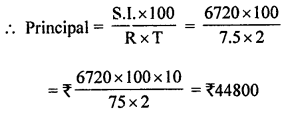## ML Aggarwal Class 8 Solutions for ICSE Maths Chapter 8 Simple and Compound Interest Ex 8.1

Question 1.
Find the simple interest on ₹4000 at 7.5% p.a. for 3 years 3 months. Also find the amount.
Solution:
Here, P (Principal) = ₹4000
R (rate of interest) = 7.5% p.a. = $$\frac{15}{2}$$% p.a
T (time) = 3 years 3 months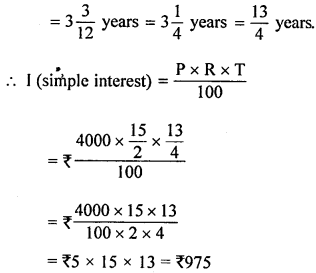Amount = P + I = ₹14000 + ₹975 = ₹4975

Question 2.
What sum of money will yield ₹170·10 as simple interest in 2 years 3 months at 6% per annum?
Solution:
Here, I = ₹170·10, T = 2 years 3 months
= 2$$\frac{3}{12}$$ years = 2$$\frac{1}{4}$$ years
= $$\frac{9}{4}$$ years
R = 6%Question 3.
Find the rate of interest when ₹800 fetches ₹130 as simple interest in 2 years 6 months.
Solution:
Here, P = ₹800, I = ₹130
T = 2 years 6 months = 2$$\frac{6}{12}$$ years= $$\frac{13}{2}$$ % p.a.= 6·5% p.a.

Question 4.
Find the time when simple interest on ₹3·3 lakhs at 6·5% per annum is ₹75075.
Solution:
Here, P = 3·3 lakhs = ₹3·3 × 100000 = ₹330000
R = 6·5% per annum
I= ₹75075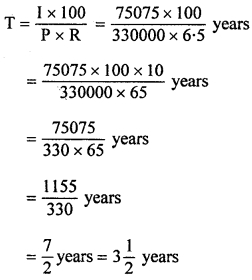Question 5.
Find the sum of money when
(i) simple interest at 7$$\frac{1}{4}$$% p.a. for years is ₹2356·25
(ii) final amount is ₹ 11300 at 4% p.a. for 3 years 3 months.
Solution:
(i) Here, I = ₹2356·25(ii) Amount (A) = ₹11300
Rate (R) = 4% p.a.
Time (T) = 3 years 3 months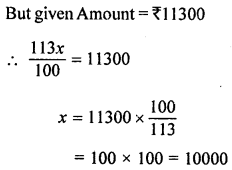Hence principal (P) = ₹10000

Question 6.
How long will it take a certain sum of money to triple itself at 13$$\frac{1}{3}$$% per annum simple interest?
Solution:
Let the sum of money = ₹ x
Amount = 3 × ₹ x = ₹ 3x
Interest = Amount – Principal = ₹3x – ₹x = ₹2x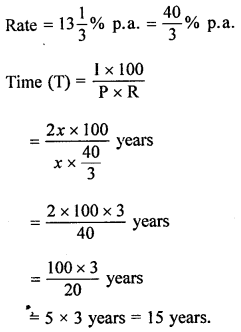Question 7.
At a certain rate of simple interest ₹4050 amounts to ₹4576·50 in 2 years. At the same rate of simple interest, how much would ₹1 lakh amount to in 3 years?
Solution:
Here, P = ₹4000, A = ₹4576·50,
T = 2 years
I = A – P = ₹4576·50 – ₹4050 = ₹526.50
Let the rate of simple interest be R% per annum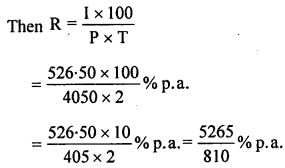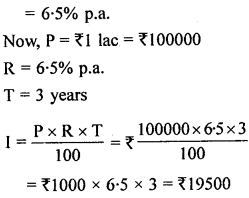Amount = Principal + Interest = ₹100000 + ₹19500 = ₹119500

Question 8.
What sum of money invested at 7.5% p.a. simple interest for 2 years produces twice as much interest as ₹9600 in 3 years 6 months at 10% p.a. simple interest?
Solution:
In first case,
Principal (P1) = ₹9600
Rate (R1) = 10%
Period (T) = 3 years 6 months = $$3 \frac{1}{2}=\frac{7}{2}$$ years
S. Interest = $$\frac{P R T}{100}=\frac{9600 \times 10 \times 7}{100 \times 2}$$ = ₹3360
In second case,
S. Interest = ₹3360 × 2 = ₹6720
Rate (R) = 7.5% p.a.
and Period (T) = 2 years